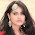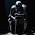## Saturday, 13 July 2013

### CBSE Class 7 - Science - CH13: Motion and Time (MCQs)

Motion and Time
MCQs

Q1: Which of the following instrument measures time with the most accuracy?

(a) sun dial
(b) sand clock
(c) quartz clock
(d) mechanical clock with gears

Q2: The basic unit of speed is:

(a) km/min
(b) m/min
(c) km/h
(d) m/s

Q3: A faster moving object covers:

(a) Less distance in more time
(b) More distance in more time
(c) Less distance in shorter time
(d) More distance in shorter time

Q4: 36 km/hr can also be expressed as:

(a) 10 m/s
(b) 3.6 m/s
(c) 100 m/s
(d) 36 m/s

Q5: If the an object moving along a straight line, keeps changing its speed then,

(a) The motion is said to be a uniform motion
(b) The motion is said to be a non uniform motion
(c) The object is said to be stationary
(d) The motion is said to be a fast motion

Q6: The motion that repeats itself after regular intervals of time can be ___ (Find the incorrect option).

(a) To and Fro Motion
(b) Circular Motion
(c) Rectilinear Motion
(d) Periodic Motion

Q7: The metallic ball in a pendulum is called as _______

(a) Gong
(b) Fork
(c) Rod
(d) Bob

Q8: Which of the following relations is correct?

(a) Speed = Distance × Time
(b) Speed = Distance ÷ Time
(c) Speed = Time ÷ Distance
(d) Speed = 1/(Distance × Time)

Q9: Which of the following is a scalar quantity?

(a) Distance
(b) Displacement
(c) Velocity
(d) Force

Q10: The meter that is used to measure the distance moved by the vehicle is known as _____

(a) Speedometer
(b) Odometer
(c) Chronometer
(d) Ammeter

Answers:
1: (c) quartz clock
2: (d) m/s
3: (d) More distance in shorter time
4: (a) 10 m/s
5: (b) The motion is said to be a non uniform motion
6: (c) Rectilinear Motion

7: (d) Bob
8: (b) Speed = Distance ÷ Time
9: (a) Distance

10: (b) Odometer#### 16 comments:

1.1.GunaSekaran Muthukrishnan you are wrong

2.Guna,1km=1000meters

2.hey GunaSekaran Muthukrishnan you are wrong

1.2.3.4.5.question no 10 (a)

6.no it is odometer. speedometer is used for finding the speed of the object. odometer shows the distance.
Thanks
Regards Aryan

1.correct...

2.correct...

7.This comment has been removed by the author.

8.very good exercise.BUT SHOULD PLACE FOR MARKING ANSWERS.OTHERWSE THE APP IS GOOD

9.Q.No.4.Ans. 36×5÷18=10 m/s.

10.Q.No.4.Ans. 36×5÷18=10 m/s.

We love to hear your thoughts about this post!